# Calculate Latency and Doppler in a Satellite Scenario

This example shows how to model a GPS satellite constellation from a SEM almanac, analyze access between the satellites and a ground station, and calculate the latency and the doppler frequency between the satellites and the ground station.

### Create a Satellite Scenario

Create a satellite scenario with a start time of 11-January-2020 2:50:00 PM UTC and a stop time 3 days later. Set the simulation sample time to 60 seconds.

```startTime = datetime(2020,1,11,14,50,0); stopTime = startTime + days(3); sampleTime = 60; % seconds sc = satelliteScenario(startTime,stopTime,sampleTime);```

### Add a Satellite to the Scenario

Add a satellite to the scenario from the `gpsAlmanac.txt` SEM almanac file.

`sat = satellite(sc,"gpsAlmanac.txt");`

The default orbit propagator when creating satellites using SEM almanac is `gps`. This can be verified by observing the `OrbitPropagator` property. Additionally, the output of the `orbitalElements` function contains GPS satellite-specific information.

`orbitProp = sat(1).OrbitPropagator`
```orbitProp = "gps" ```
`elements = orbitalElements(sat(1))`
```elements = struct with fields: PRN: 1 GPSWeekNumber: 2087 GPSTimeOfApplicability: 589824 SatelliteHealth: 0 SemiMajorAxis: 2.655951847442722e+07 Eccentricity: 0.009273052215576 Inclination: 56.066459655761712 GeographicLongitudeOfOrbitalPlane: -40.477838516235359 RateOfRightAscension: -4.616595106199388e-07 ArgumentOfPerigee: 43.383057117462180 MeanAnomaly: 1.726436662673951e+02 Period: 4.307658596414280e+04 ```

### Add a Ground Station

Add the Madrid Deep Space Communications Complex as the ground station of interest, and specify its latitude and longitude.

```name = "Madrid Deep Space Communications Complex"; lat = 40.43139; % degrees lon = -4.24806; % degrees gs = groundStation(sc,Name=name,Latitude=lat,Longitude=lon);```

### Add an Access Analysis

Add access analysis between the GPS satellites and Madrid ground station, which determines when the ground station is visible to the satellites. This determines when latency and doppler should be calculated.

```ac = access(sat,gs); [acStatus,time] = accessStatus(ac);```

### Visualize Scenario

Visualize the scenario by launching a satellite scenario viewer. Set `ShowDetails` of the viewer to false to ensure the visualization is not cluttered. Set the camera position to bring all satellites that have access to the ground station into view.

```v = satelliteScenarioViewer(sc,ShowDetails=false); campos(v, ... 29, ... % Latitude, degrees -19, ... % Longitude, degrees 7.3e7); % Altitude, degrees```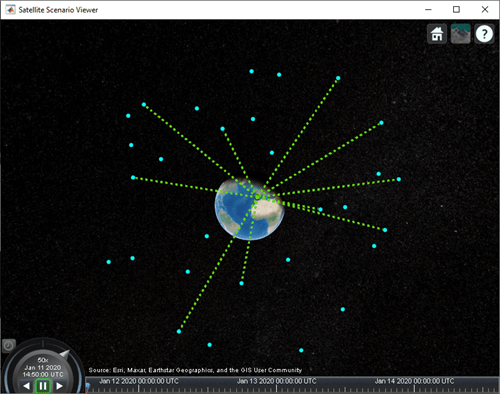### Calculate Latency and Velocity

Calculate the latency between the GPS satellites and the Madrid ground station. Also, calculate the velocity along the line between each satellite and the ground station. A positive value indicates that the satellite is moving towards the ground station, and a negative value indicates the satellite is moving away from the ground station.

```% Calculate azimuth, elevation, and range from each satellite to the ground % station. [az,el,r] = aer(sat,gs); % Calculate latency. c = physconst("Lightspeed"); latency = r/c; % Calculate the velocity of each satellite in its respective % North/East/Down (NED) frame. Physically, this is the ECEF velocity, % represented in NED frame of the satellite. Therefore, the relative % velocity with respect to the ground station is also the same. [~,satV] = states(sat,CoordinateFrame="geographic"); % Calculate the direction of the ground station with respect to each % satellite in its respective NED frame. satV is a 3-dimensional array, % wherein the first dimension represents the cartesian component, second % dimension represents the time sample, and third dimension represents the % satellite. Therefore, compute the direction also as a 3-dimensional % array, wherein the first dimension has a size of 1, the second dimension % represents the time sample, and the third dimension corresponds to the % satellite. To do this, az and el must also be re-formatted such that the % first dimension, which represents the satellite becomes the third % dimension. You can use permute to re-format the arrays as described. dir = cat(1,permute(cosd(el).*cosd(az),[3 2 1]), ... permute(cosd(el).*sind(az),[3 2 1]), ... permute(-sind(el),[3 2 1])); % Calculate the velocity along the line between the ground station and each % satellite. This velocity determines the doppler frequency corresponding % to each satellite. Re-format this velocity such that the first dimension % corresponds to the satellite. dopV = permute(dot(satV,dir),[3 2 1]); % Set the latency and doppler velocity to NaN whenever access status is % false because these quantities are irrelevant when there is no access. latency(~acStatus) = NaN; dopV(~acStatus) = NaN;```

Plot the latency corresponding to the first satellite. You may choose to plot the latency corresponding to all satellites. However, this example plots it only for the first satellite to serve as a demonstration and to reduce plot clutter.

```plot(time,latency(1,:)) xlim([sc.StartTime sc.StopTime]) title("Latency vs. Time") xlabel("Simulation Time") ylabel("Latency (ms)") grid on```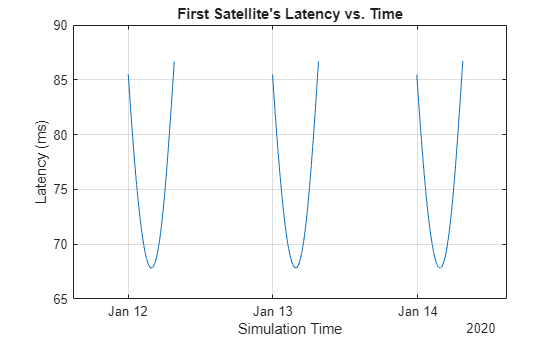Plot the velocity of the first satellite along the line between itself and the ground station.

```plot(time,dopV(1,:)) xlim([sc.StartTime sc.StopTime]) title("Velocity of First Satellite Along the Line Between First Satellite and GS") xlabel("Simulation Time") ylabel("Velocity (m/s)") grid on```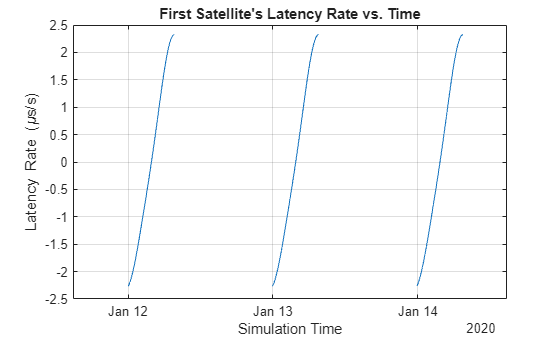### Calculate Doppler Frequency from Velocity

The doppler frequency is calculated from the following equation:

${\mathit{f}}_{\mathit{o}}=\left(\frac{\mathit{c}±{\mathit{v}}_{\mathit{r}}}{\mathit{c}±{\mathit{v}}_{\mathit{s}}}\right){\mathit{f}}_{\mathit{e}}$,

where $\mathit{c}$ is the speed of light in m/s,

${\mathit{v}}_{\mathit{r}}$ is the speed, in m/s, of the receiver relative to the medium, added to $\mathit{c}$ if the receiver is moving towards the source, subtracted if the receiver is moving away from the source,

${\mathit{v}}_{\mathit{s}}$ is the speed, in m/s, of the source relative to the medium, added to $\mathit{c}$ if the source is moving away from the receiver, subtracted if the source is moving towards the receiver,

${\mathit{f}}_{\mathit{e}}$ is the emitted frequency, in Hz, and

${\mathit{f}}_{\mathit{o}}$ is the observed frequency, in Hz.

In this example, we consider the observed frequency from the standpoint of a receiving ground station. In that case, ${\mathit{v}}_{\mathit{r}}$ is 0. We also consider a C band frequency of 5 GHz.

```fe = 5e9; % emitted frequency in Hz fShift = (((c ./ (c-dopV)) * fe) - fe); % doppler shift in Hz```

Plot the doppler shift corresponding to the first satellite.

```plot(time,fShift(1,:)/1e3) % plot kHz xlim([sc.StartTime sc.StopTime]) title("Doppler Shift Corresponding to First Satellite vs. Time") xlabel("Simulation Time") ylabel("Doppler Shift (kHz)") grid on```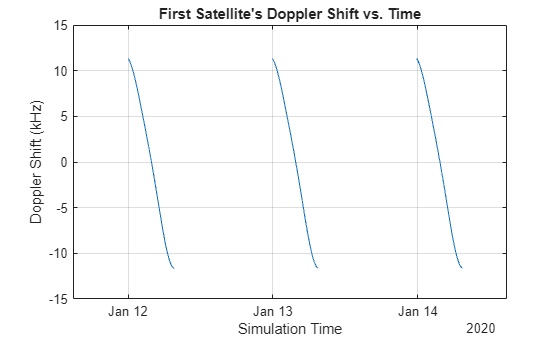### Calculate Rates of Change for Latency and Doppler

Satellite communications links need to be designed to track varying latencies and Doppler frequencies. Thus, it is important to calculate these quantities. Plot these quantities corresponding to the first satellite.

```latencyRate = diff(latency,1,2)/sampleTime; plot(time(1:end-1),latencyRate(1,:)) % seconds/second xlim([sc.StartTime time(end-1)]) title("Latency Rate of Change Corresponding to First Satellite") xlabel("Simulation Time") ylabel("Latency Rate of Change (ms/s)") grid on```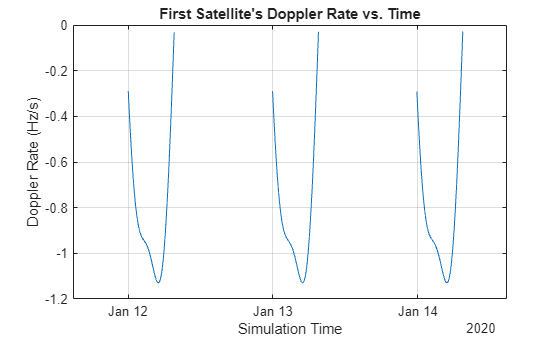```dopplerRate = (diff(fShift,1,2)/sampleTime); plot(time(1:end-1),dopplerRate(1,:)) xlim([sc.StartTime time(end-1)]) title("Doppler Rate of Change Corresponding to First Satellite") xlabel("Simulation Time") ylabel("Doppler Rate of Change (Hz/s)") grid on```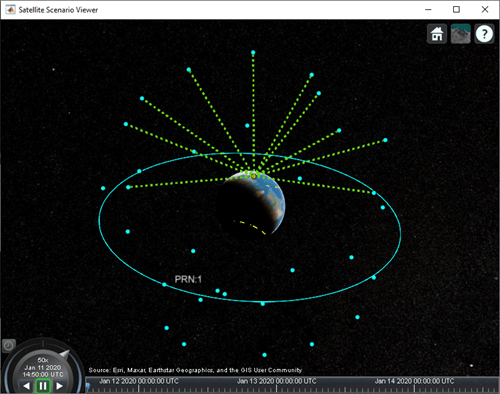Show the name and orbit of the first satellite and plot its future ground track, or lead time, over 12 hours. Dashed yellow shows the future ground track, and solid yellow shows the past ground track.

```sat(1).ShowLabel = true; show(sat(1).Orbit); groundTrack(sat(1), ... LeadTime=12*3600); % seconds campos(v,84,-176,7.3e7);```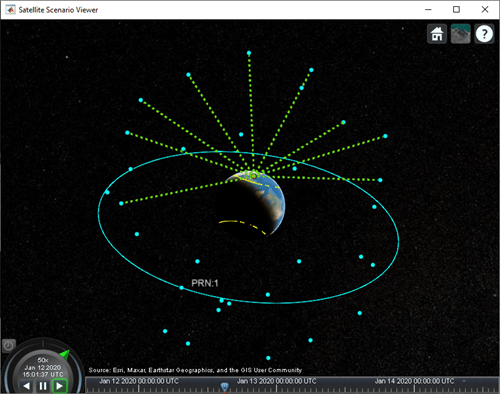Play the scenario with the satellites and ground station.

`play(sc)`